# Power BI 地理分析之形状地图

159_模型_Power BI 地理分析之形状地图

## 一、背景

• 省级市场共计：34 个，已开拓：34 个；占比：100.0%。

• 地市级市场共计：370 个，已开拓：214 个；占比：57.8%。

• 区县级市场共计：2875 个，已开拓：356 个；占比：12.4%。

Power BI 公共 web 效果：https://demo.jiaopengzi.com/pbi/159-full.html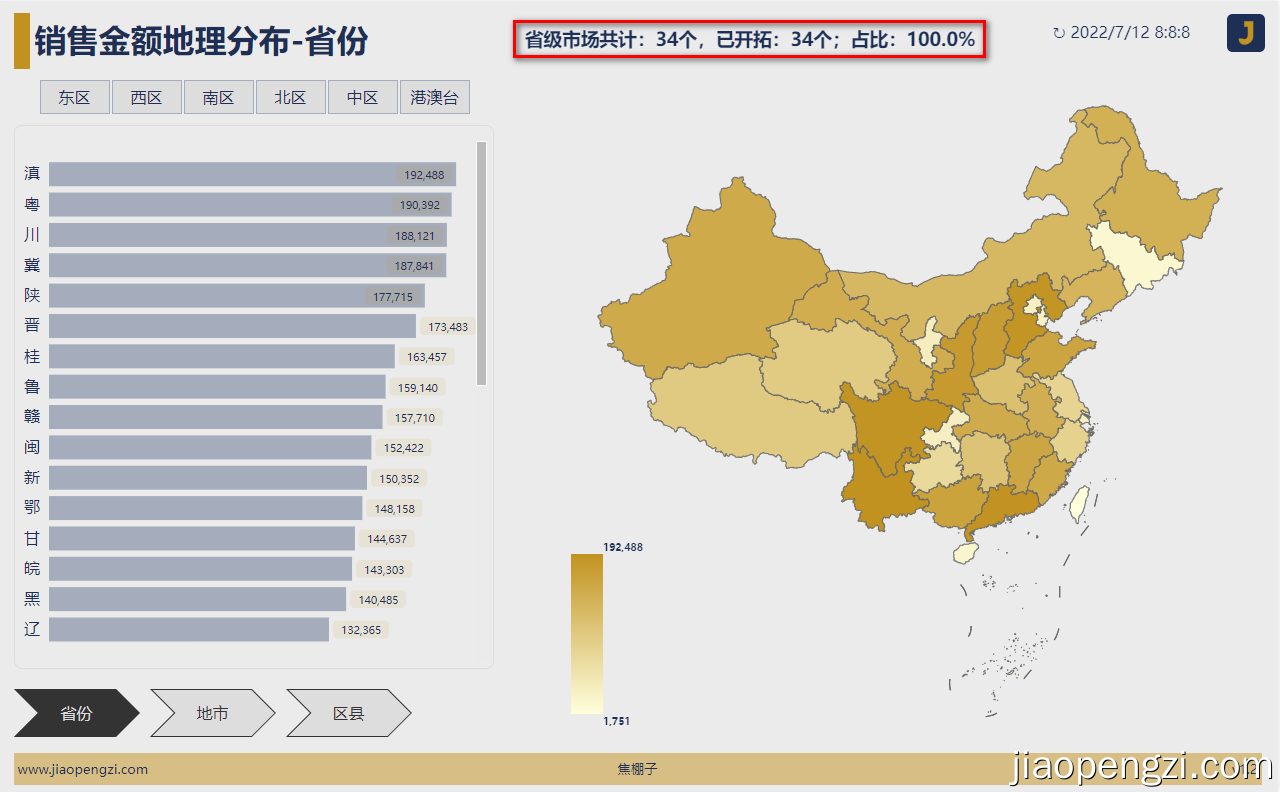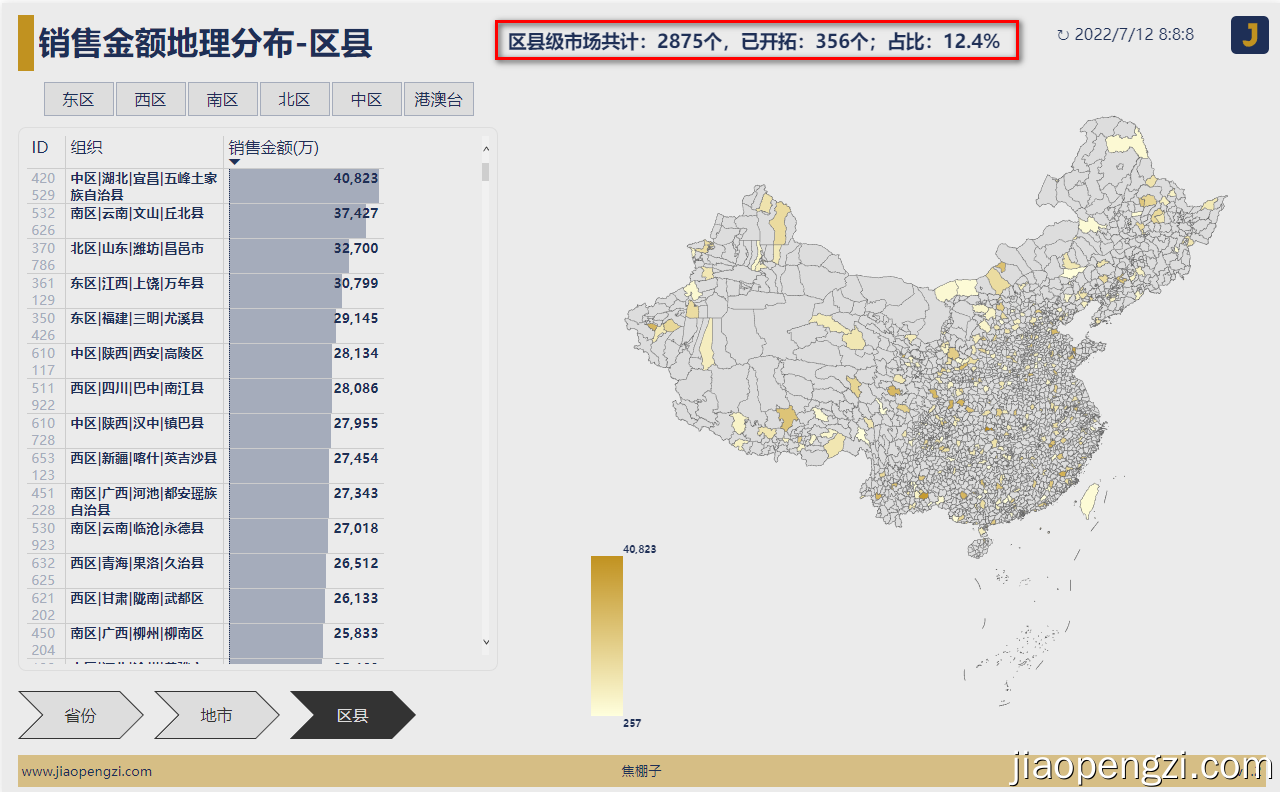## 二、模型设计

### 1、地图数据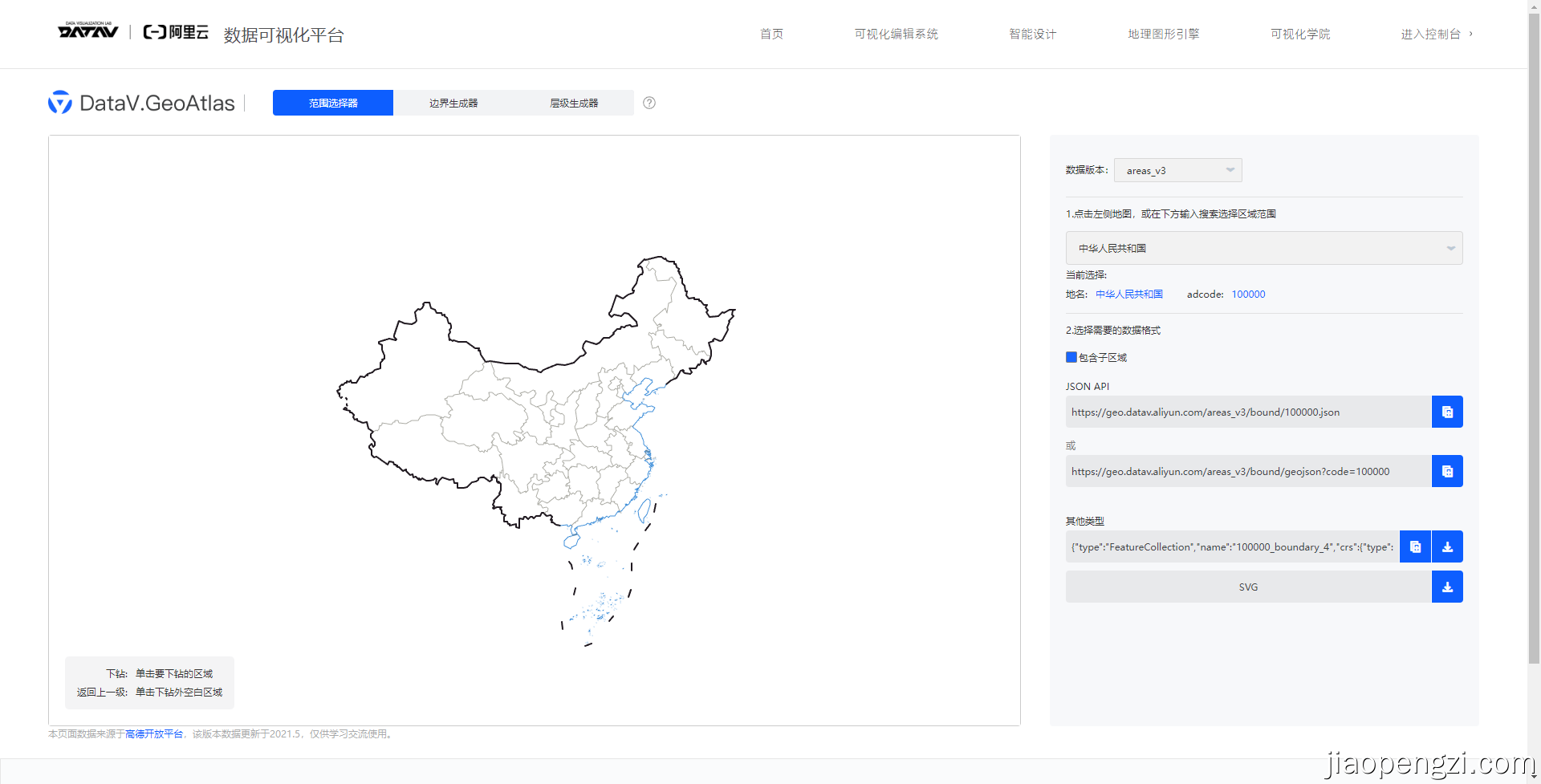GeoJSON 转换为 TopoJSON 我们使用开源的工具 mapshaper (https://github.com/mbloch/mapshaper) 转换即可。数量不是特别多的话可以使用可视化的方式转换将 GeoJson 格式数据导入到 https://mapshaper.org/ 导出即可。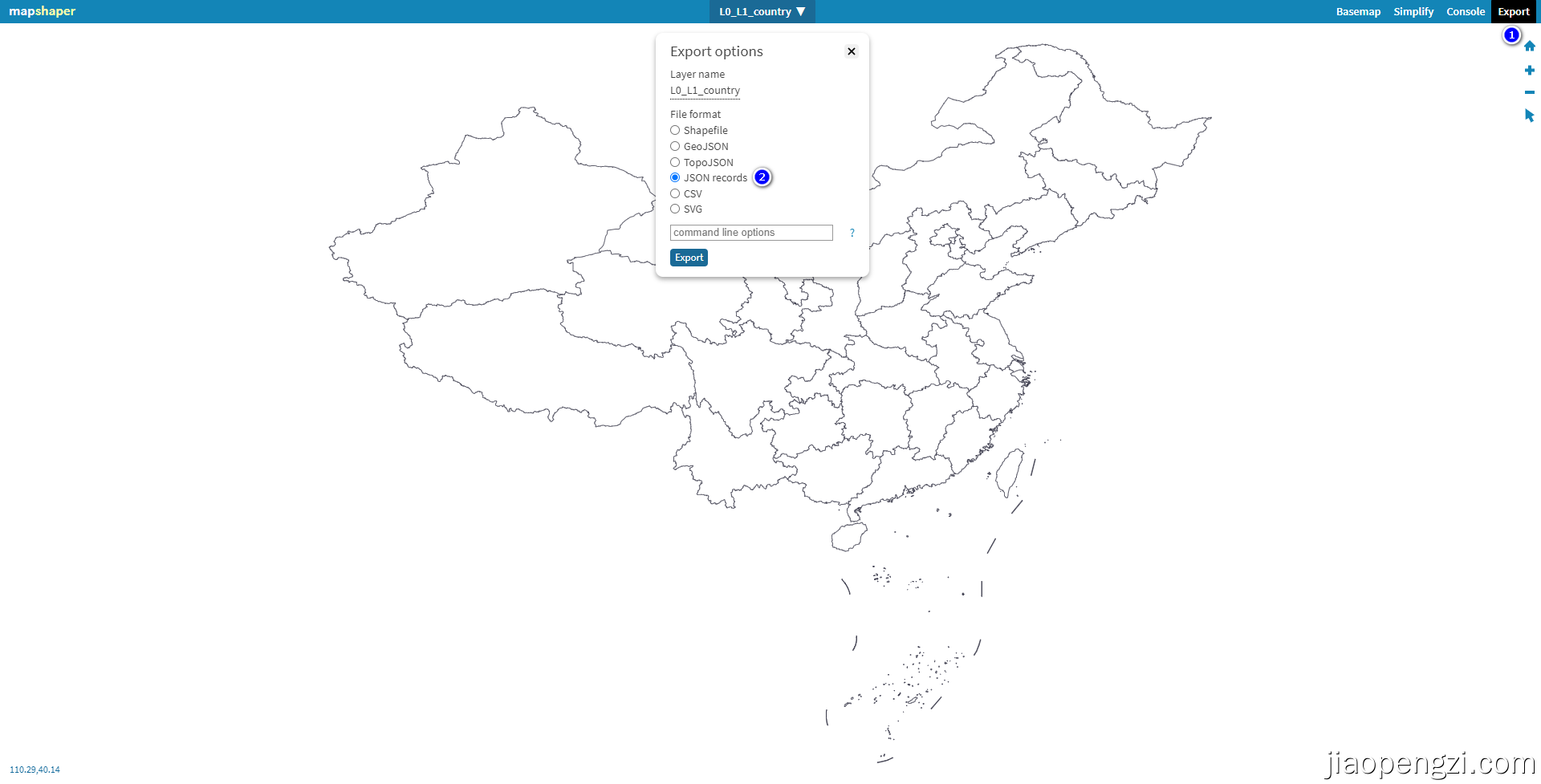``npm install -g mapshaper``

``mapshaper-gui``

``mapshaper -i D:\Desktop\map\map_data\geo\country\L0_L1_country.json -o D:\Desktop\map\map_data\topo\country\L0_L1_country.json format=topojson``

Python 配合 mapshaper 可以批量地拆分和转换 GeoJSON 数据。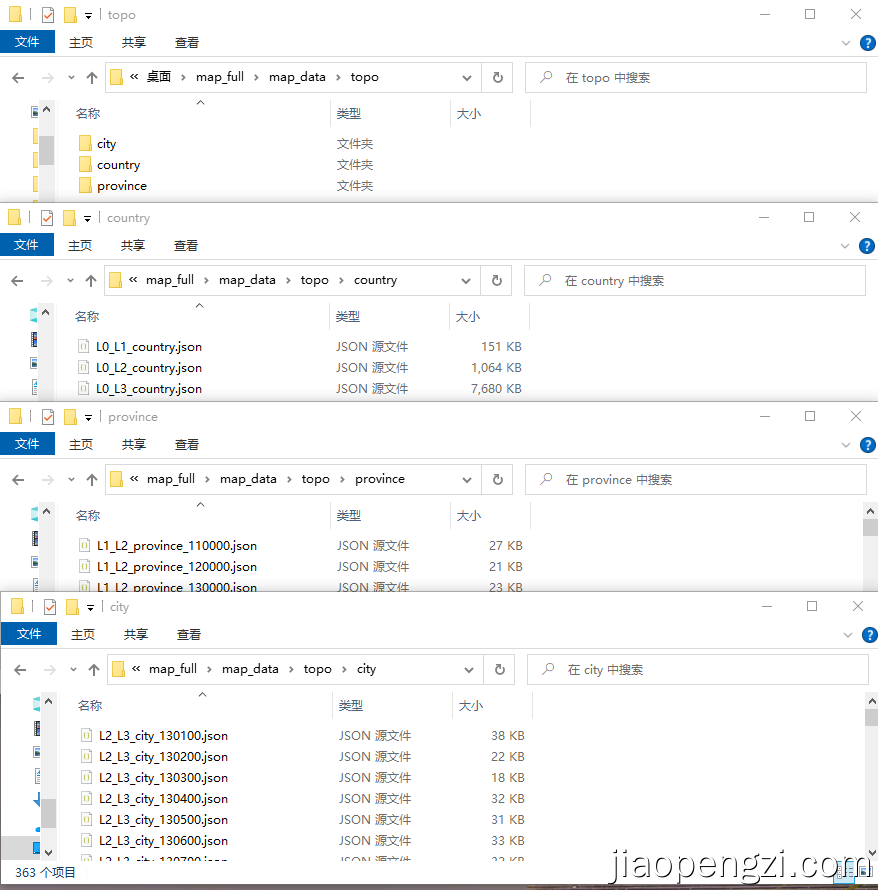# L0-全国 L1-省份 L2-地市 L3-区县 文件夹 文件命名规则
1 country L0_L1_country
2 country L0_L2_country
3 country L0_L3_country

### 2、区域维度数据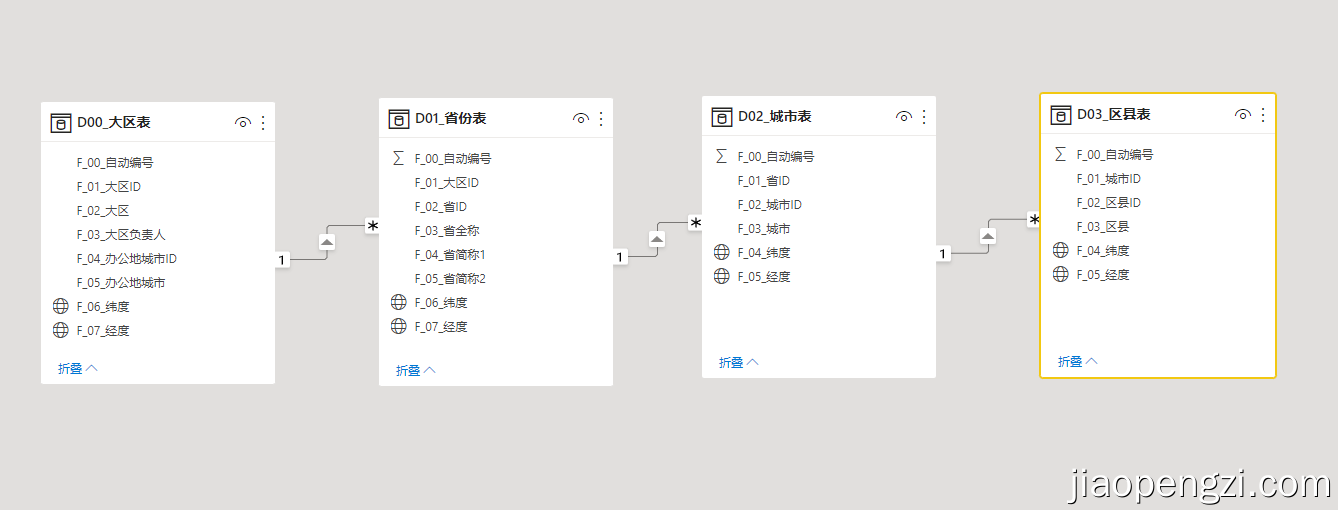### 3、模型关系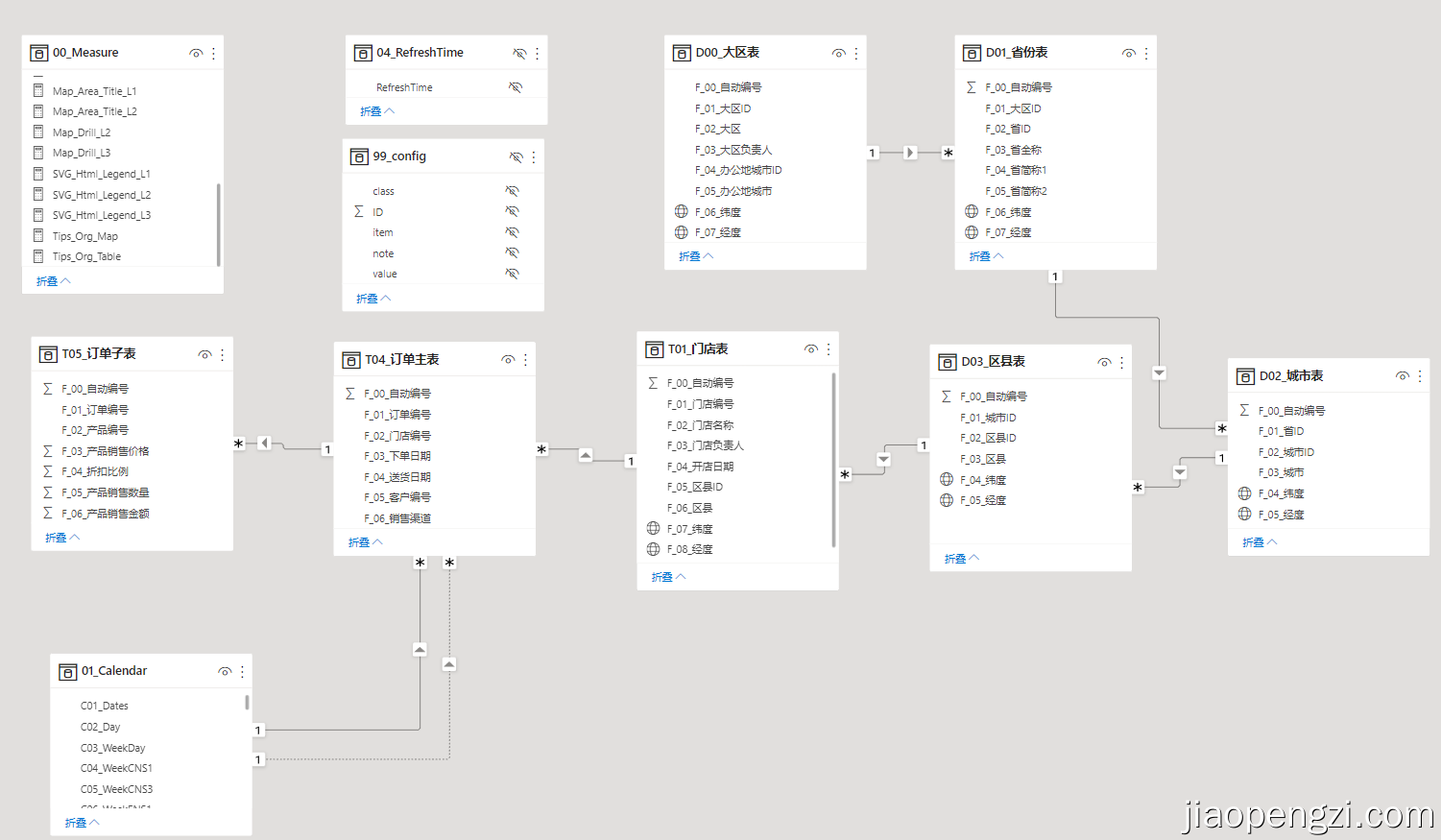## 三、度量值及可视化

### 1、度量值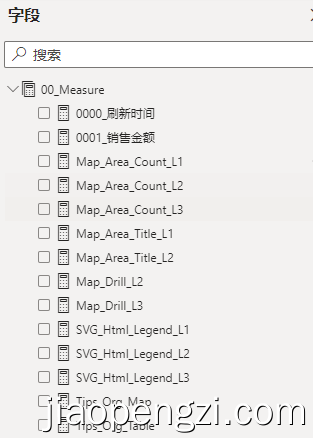Ⅰ、基础的业务度量值：0001_销售金额。

``````0001_销售金额 =
SUM ( 'T05_订单子表'[F_06_产品销售金额] ) / 10000 ``````

Ⅱ、市场占有结论语句度量值：Map_Area_Count ；L1-L3分别代表省份、地市、区县。

``````Map_Area_Count_L1 =
VAR Table_ID =
VALUES ( 'D01_省份表'[F_02_省ID] )
VAR Table_All =
ADDCOLUMNS ( Table_ID, "@value", [0001_销售金额] )
VAR Table_Sale = FILTER( Table_All ,[@value] <> BLANK())
VAR FZ =
COUNTROWS ( Table_Sale )
VAR FM =
COUNTROWS ( 'D01_省份表' )
VAR Format_1 =
FORMAT ( DIVIDE ( FZ, FM, BLANK () ), "#0,0.0%" )
RETURN
"省级市场共计：" & FM & "个，已开拓：" & FZ & "个；占比：" & Format_1``````

Ⅲ、数据向下钻取后标题名称：Map_Area_Title ；L1-L2分别代表省份、地市。

``Map_Area_Title_L1 = SELECTEDVALUE('D01_省份表'[F_05_省简称2])``

Ⅳ、数据向下钻取后，形状地图的色彩饱和度度量值：Map_Drill ；L2-L3分别代表地市、区县。这里需要兼容一个形状地图不显示没有数据的区域，那么我们需要构造下钻后的所有区域的数据，没有的数据就是 0 。

``````Map_Drill_L2 =
VAR PARENT_ID =
SELECTEDVALUE ( 'D02_城市表'[F_01_省ID] )
VAR TABLEY =
'D02_城市表',
"@VALUE",
VAR p = [F_01_省ID]
RETURN
IF ( p = PARENT_ID, '00_Measure'[0001_销售金额] + 0, '00_Measure'[0001_销售金额] )
)
VAR TABLEZ =
FILTER ( TABLEY, [F_01_省ID] = PARENT_ID )
RETURN
SUMX ( TABLEZ, [@VALUE] )``````

Ⅴ、由于 Power BI 目前是图例来显示数据的多少的，我们用 SVG 构造一个图例：SVG_Html_Legend ;L1-L3分别代表省份、地市、区县。

``````SVG_Html_Legend_L1 =
VAR table_all =
CALCULATETABLE ( 'D01_省份表', ALL ( 'D01_省份表'[F_02_省ID] ) )
ADDCOLUMNS ( table_all, "@value", [0001_销售金额] )
VAR value_max =
FORMAT ( MAXX ( table_add, [@value] ), "#,0" )
VAR value_min =
FORMAT ( MINX ( table_add, [@value] ), "#,0" )
VAR value_ac =
FORMAT ( [0001_销售金额], "#,0" )
VAR FM =
VAR FZ =
MAXX ( table_add, [@value] ) - [0001_销售金额]
VAR path_y = 160 * FZ / FM + 20
VAR TF0 =
HASONEVALUE ( 'D01_省份表'[F_02_省ID] )
VAR TF1 =
HASONEFILTER ( 'D01_省份表'[F_04_省简称1] )
VAR SVG_start_image = "data:image/svg+xml;utf8," //SVG 图像类型头部。
VAR SVG_start_html = "<svg id='jiaopengzi' width='100' height='200' viewBox='0 0 100 200' xmlns='http://www.w3.org/2000/svg' version='1.1'>" //SVG html类型头部。
VAR SVG_end = "</svg>"//SVG 结束标签。
VAR SVG_defs = "
<defs>
<linearGradient id='jiaopengzi-linear' x1='0%' y1='100%' x2='0%' y2='0%'>
<stop offset='0%' stop-color='#FFFFDD' />
<stop offset='100%' stop-color='#C19220' />
</defs>
"
VAR SVG_content_0 = "
<rect x='0' y='20' width='32' height='160' fill='url(#jiaopengzi-linear)' />
<g fill='#1E2F56' font-family='Microsoft Yahei' font-size='10' text-anchor='start' font-weight='bold'>
<text x='32' y='20' dominant-baseline='text-after-edge'>" & value_max & "</text>
<text x='32' y='180' dominant-baseline='text-before-edge'>" & value_min & "</text>
</g>
"// 当没有筛选的时候，path 中间的 text 不出现。
VAR SVG_content_1 = "
<rect x='0' y='20' width='32' height='160' fill='url(#jiaopengzi-linear)' />
<path fill='red' stroke='' d='M0 0 L-10 -5 L-10 5 Z' transform='translate(32," & path_y & ")' />
<g fill='#1E2F56' font-family='Microsoft Yahei' font-size='10' text-anchor='start' font-weight='bold'>
<text x='32' y='20' dominant-baseline='text-after-edge'>" & value_max & "</text>
<text x='32' y='" & path_y & "' dominant-baseline='middle'>" & value_ac & "</text>
<text x='32' y='180' dominant-baseline='text-before-edge'>" & value_min & "</text>
</g>
"
VAR SVG_content1 =
IF ( TF0 * TF1, SVG_content_1, SVG_content_0 )
VAR SVG_content =
SWITCH ( TRUE (), TF0 && TF1, SVG_content_0, TF0, SVG_content_1, SVG_content_0 )
VAR SVG_html = SVG_start_html & SVG_defs & SVG_content & SVG_end
VAR SVG_image = SVG_start_image & SVG_html
RETURN
SVG_html``````

Ⅵ、最后在形状地图工具提示中增加区域层级显示。

``````Tips_Org_Map =
VAR L0 =
CALCULATE ( SELECTEDVALUE ( 'D00_大区表'[F_02_大区] ), 'D03_区县表' )
VAR L1 =
CALCULATE ( SELECTEDVALUE ( 'D01_省份表'[F_05_省简称2] ), 'D03_区县表' )
VAR L2 =
CALCULATE ( SELECTEDVALUE ( 'D02_城市表'[F_03_城市] ), 'D03_区县表' )
VAR L3 =
SELECTEDVALUE ( 'D03_区县表'[F_03_区县] )
VAR TF1 =
HASONEVALUE ( 'D01_省份表'[F_05_省简称2] )
VAR TF2 =
HASONEVALUE ( 'D02_城市表'[F_03_城市] )
VAR TF3 =
HASONEVALUE ( 'D03_区县表'[F_03_区县] )
VAR ORG =
SWITCH (
TRUE (),
TF3,
L0 & "|" & L1 & "|" & L2 & "|" & L3,
TF2,
L0 & "|" & L1 & "|" & L2,
TF1,
L0 & "|" & L1,
L0 & "|" & L1
)
RETURN
ORG``````

### 2、可视化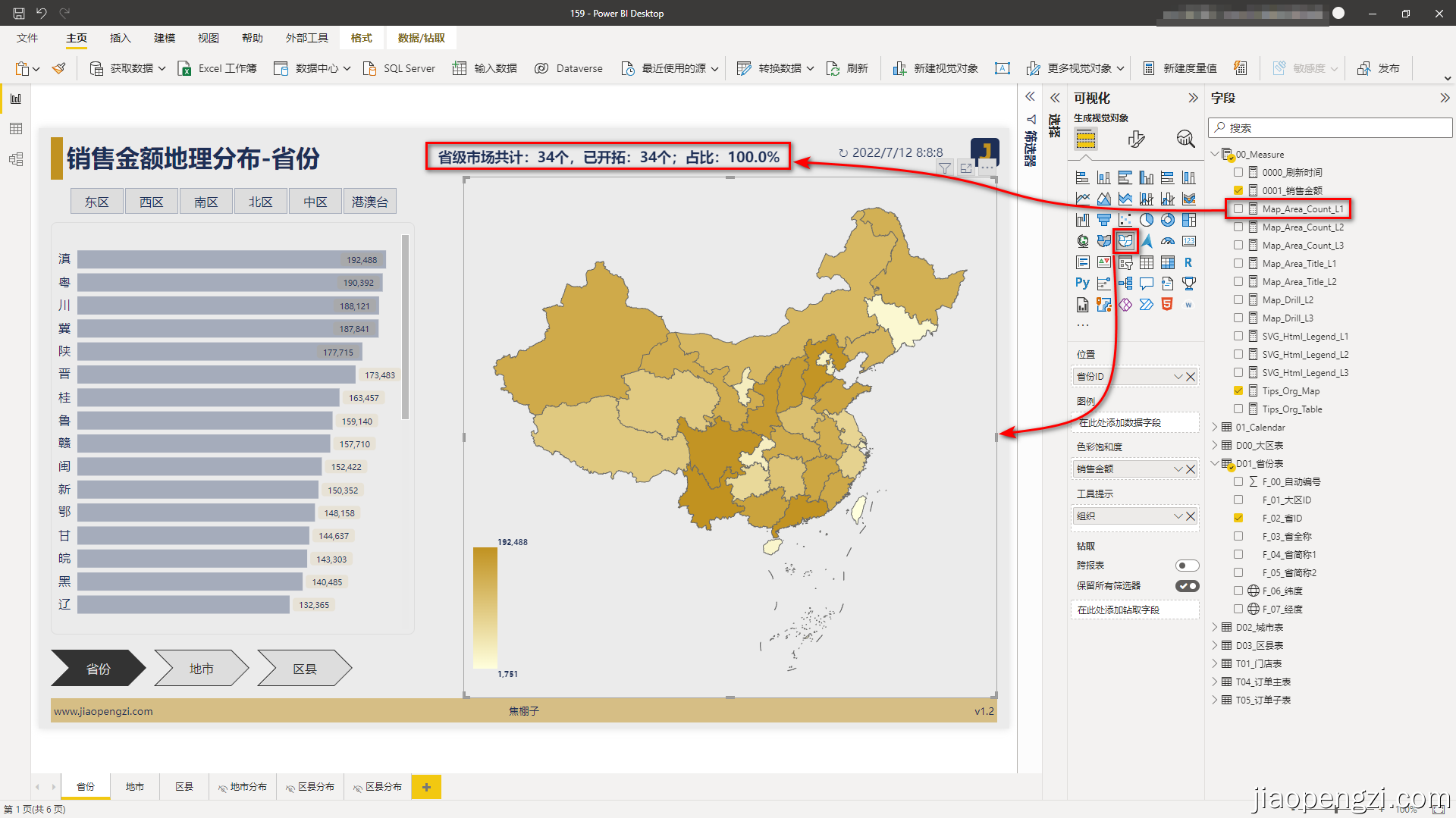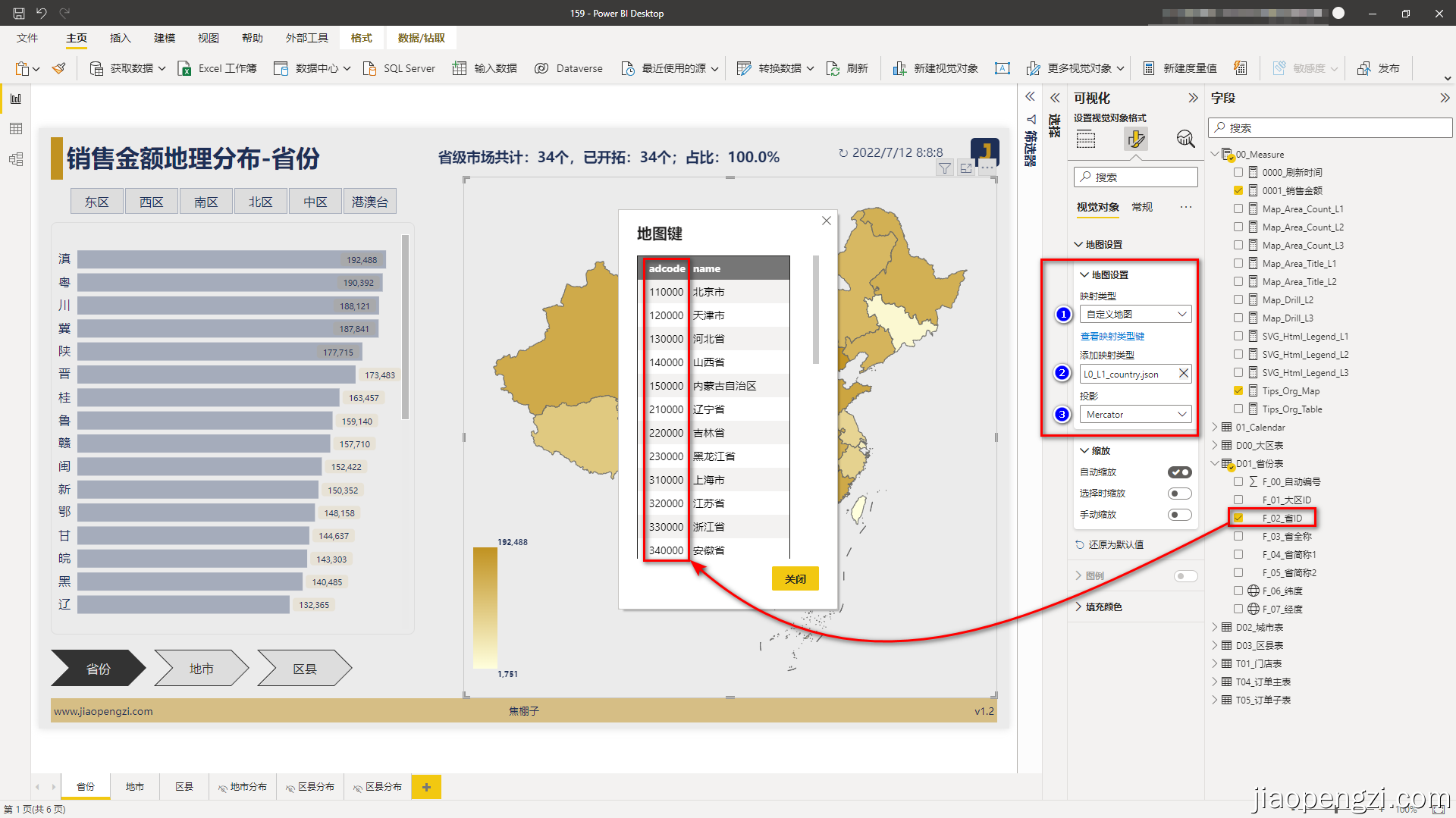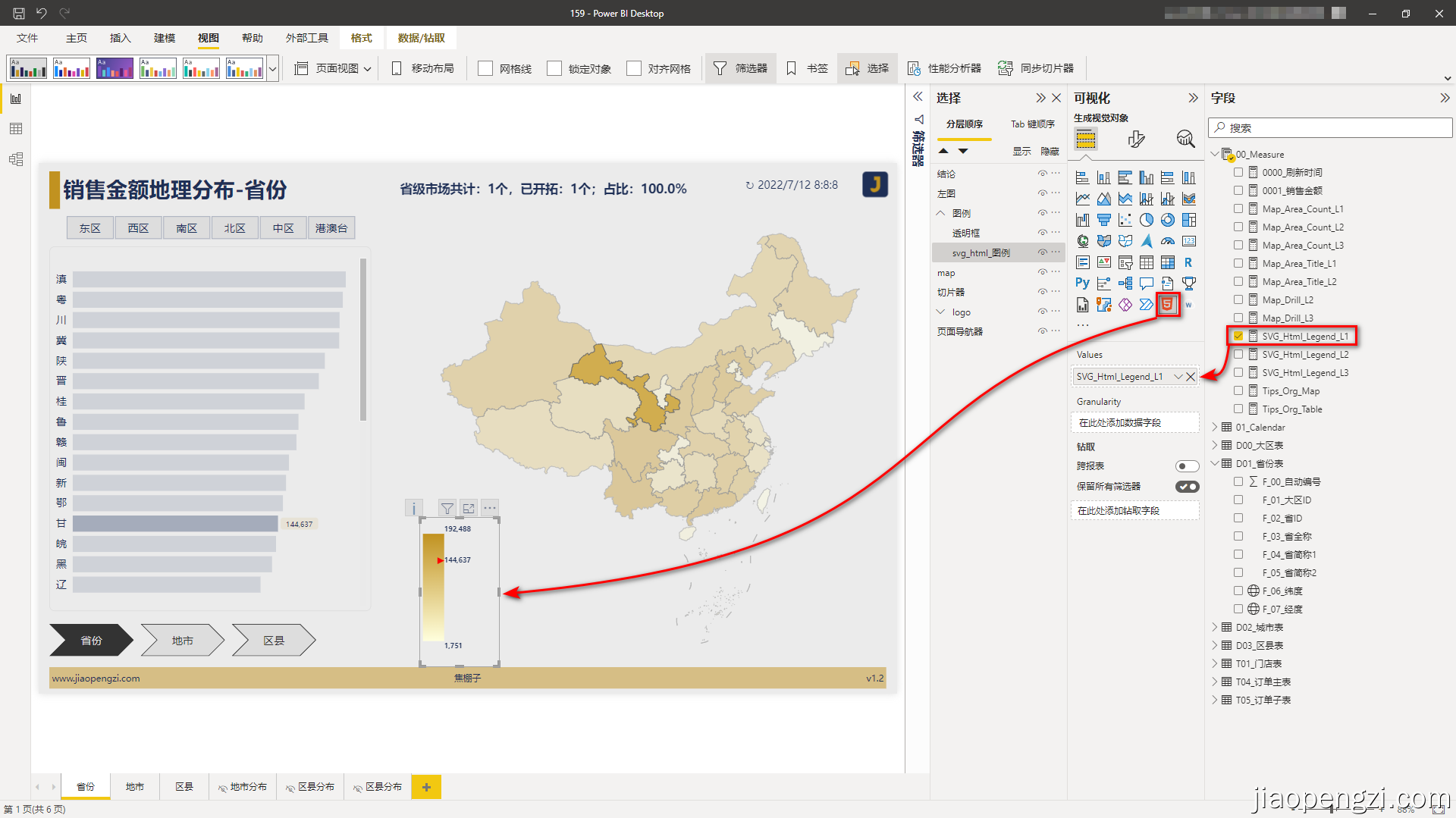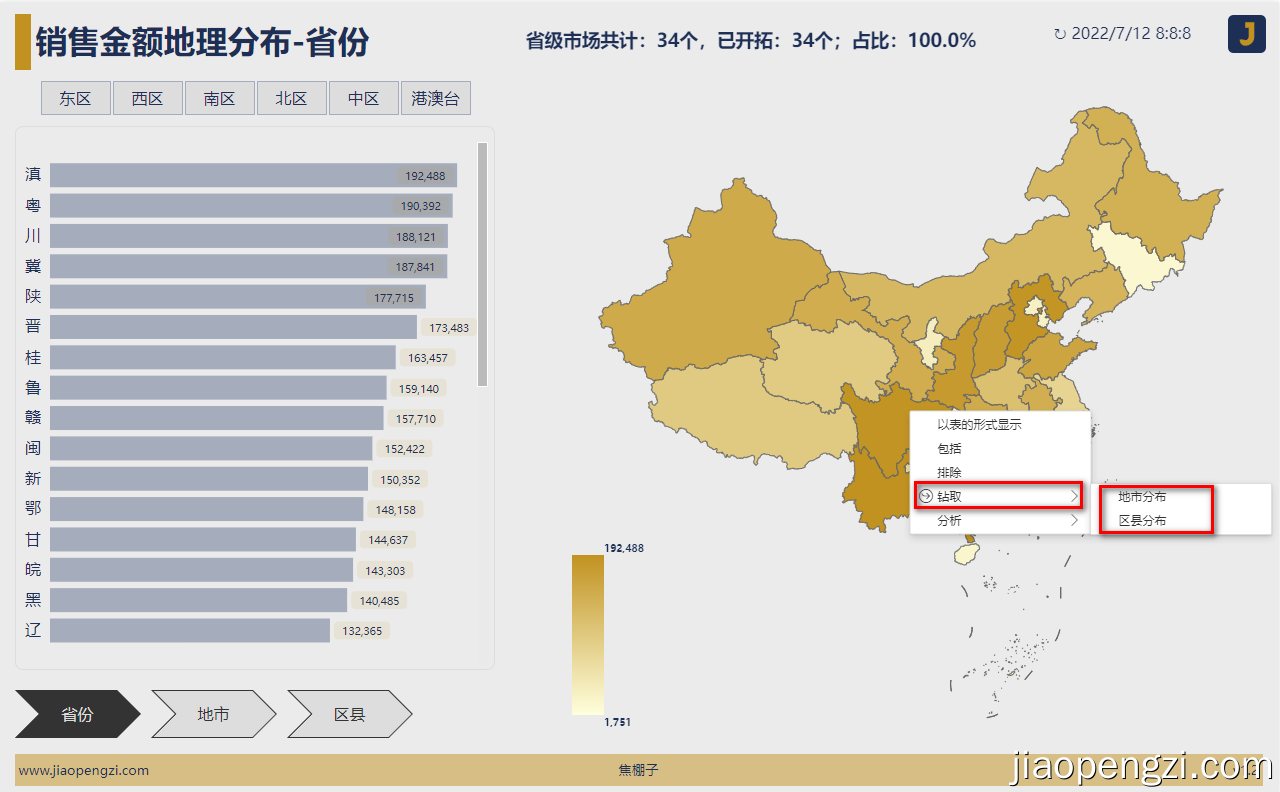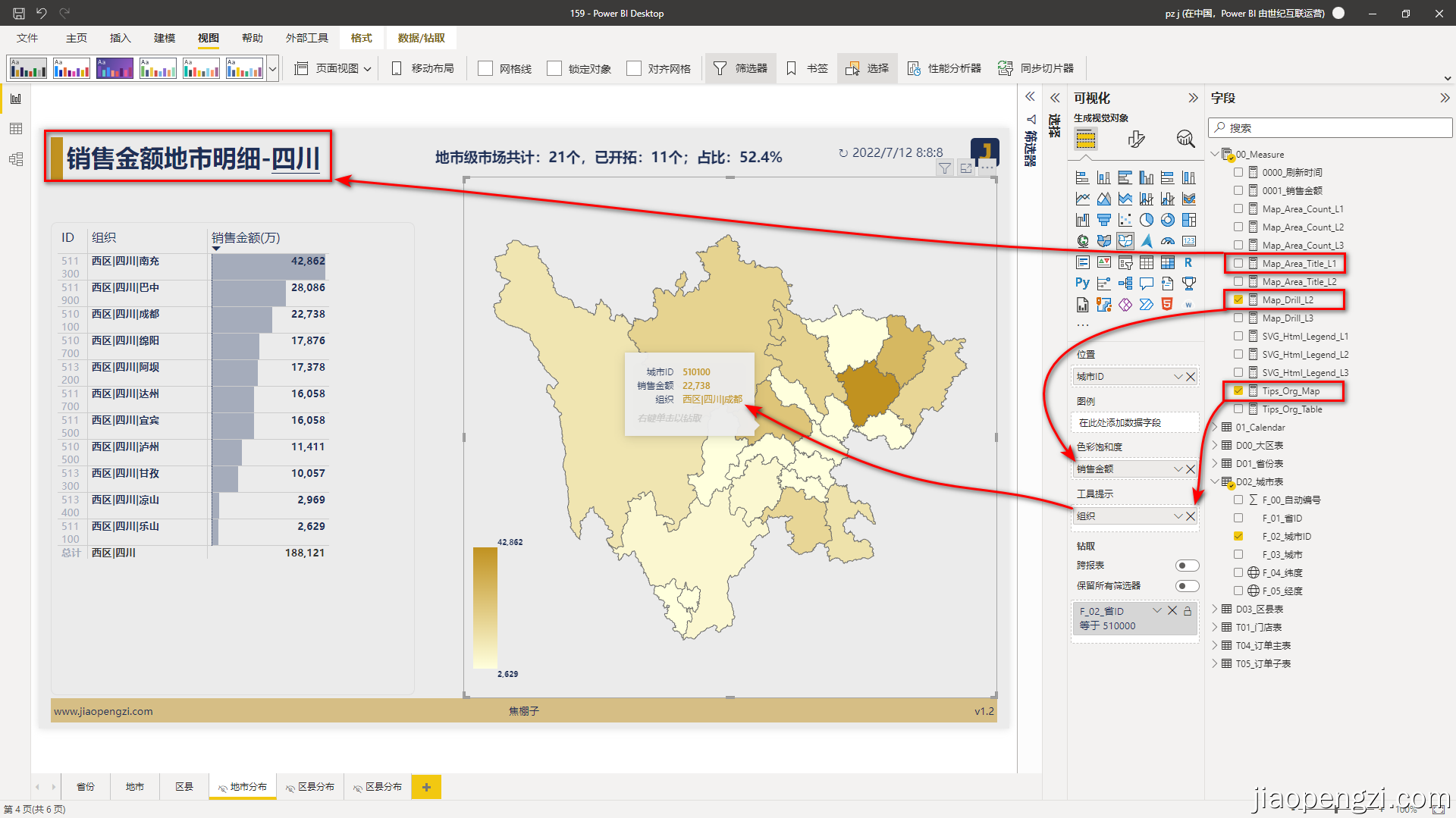## 四、总结

1、形状地图目前还是预览的状态，必须在 Power BI Desktop 中启用。 若要启用“形状地图”，请选择“文件”>“选项和设置”>“选项”>“预览功能”，然后选中“形状地图视觉对象”复选框。

2、目前形状地图还有很多不灵活的地方，比如不能在地图上显示文本标签，地图填充颜色还是写死的，不能通过 DAX 来动态驱动。

3、当前区县一级的 TopoJson 比较大，使用的时候及时保存，避免不要的麻烦。

4、由于历史原因，当前地图数据对于湾湾的数据只有一个大致的，没有更细粒度的数据。更细粒度的数据应该在不远的将来可以使用了。

## 附件下载

https://jiaopengzi.com/2816.html

## 视频课

https://jiaopengzi.com/all-course

by 焦棚子